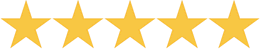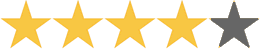A Beka Book Arithmetic 5 An information box, abundant practice of new and review concepts and facts, and daily word problems are key features of this work-text. Problem-solving strategies are scattered throughout the text to help students gain the skills necessary to be expert problem solvers. Emphasis is placed upon whole numbers, fractions, decimals, measurement and algebraic equations, basic geometric problems, and much more. Soft cover.
 Can You Count in Greek? Judy Leimbach This thorough introduction to ten different ancient number systems is a valuable resource for math enrichment or an introduction to our number system or incorporate it into any Ancient history unit.Price thisChallenge Math A unique new resource for children gifted in math. Your child will love the fascinating true stories that tie math and science together and will be challenged by over 1000 problems at three levels of difficulty. Parents will appreciate the easy to learn instructional format that makes teaching and learning enjoyable. Contains 350 pages and twenty chapters in astronomy, statistics, probability, algebra, physics, and more. Allows children to see the wondrous side of math and its important role in today's world. A great way to enrich your curriculum.Price thisBorenson & Associates Hands-on Equations Learning System Mary Pride says: ''Here's something really new-a hands-on approach to algebraic linear equations! You know what those are: fun stuff like 2x -2(-x+4)=x +(-2). With Hands-On Equations, learning pre-algebra and solving simple linear equations can be as fun and easy as playing a game.'' This patented teaching method empowers the teacher to successfully present essential algebraic concepts to students in grades 3 to adult. It does not require any algebraic prerequisites. Its system provides students with an intuitive understanding of the most fundamental algebraic concepts and makes algebraic linear equations accessible to 3rd grade gifted students, 4th grade average students, and 5th grade LD students. Hands-On Equations consists of: manuals for Levels I, II, & III; a student kit of game pieces with flat laminated balance; a set of worksheets; and an answer key.Price thisKey to Algebra Books Julie King Key to Workbooks offer a positive alternative to large, expensive textbooks which students often find complicated and intimidating. Only one concept is presented per page, so students have time to understand new ideas without being overwhelmed. Hand-written examples and exercises make work seem less formal. Vocabulary and reading levels are simple. Instructions are clear and direct. Each book is a complete text-workbook. Students develop understanding by solving equations & inequalities intuitively before formal solutions are introduced. a Full year's course that stands entirely on its own.(5)
1 ReviewPrice thisBob Jones University Press Math 5 Emphasizes the understanding of subtraction, multiplication, and division of fractions using manipulatives. Includes an optional pre-algebra chapter, which expands concepts previously taught. Develops reasoning and judgment skills through a variety of estimating and problem-solving activities. Teacher's Edition contains 165 lessons divided into 18 chapters. Includes short practice and systematic review activities to aid in the retention of concepts. Provides additional information about memorizing facts, bulletin board ideas, and mental math computations. Teacher's Edition: 942 pages; two spiral binders. Student Text presents activities using an aviation theme with Mandy Newton, a nursing student, and her dog, Rotor. Student Text: 416 pages; hardbound; full color.(5)
1 Review

 Saxon Math 54 Excellent K-12 textbook and workbook math program. Students will generally work independently. Uses an effective incremental learning model.(4.2)
4 ReviewsPrice thisMath By Kids Written by Pennsylvania Homeschoolers. This unusual book of word problems was written by homeschool students of all ages. Arranged by categories - Math Problems About Food, Working and Earning, Mystery Numbers, Etc. Problems are listed from easiest to hardiest.